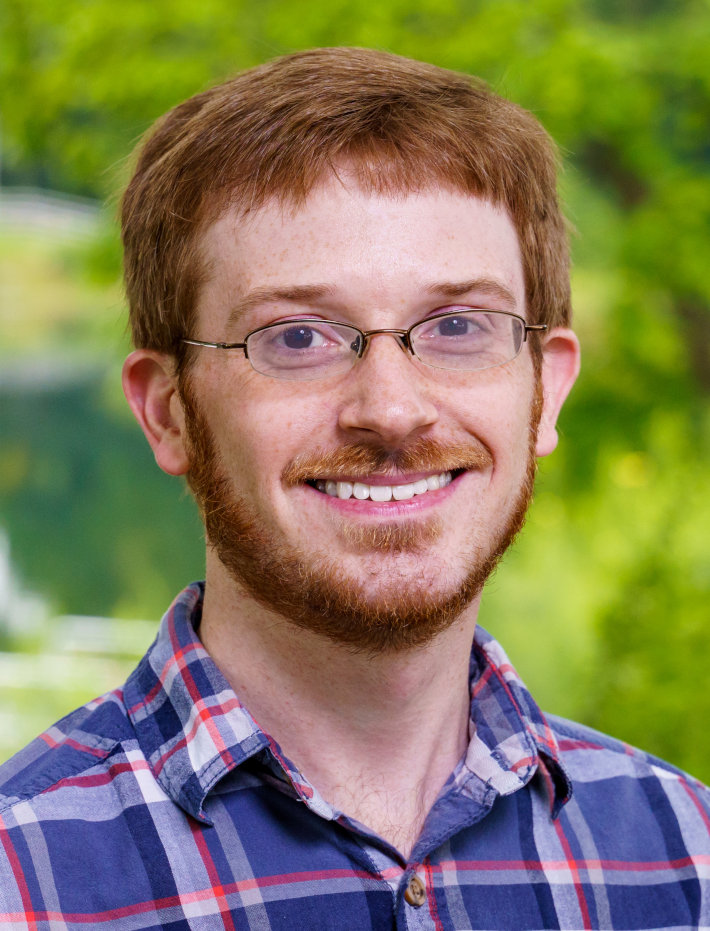Kyle Claassen
Assistant Professor of Mathematics

Office: Moench FL104
Office Hours (Fall 2023): MTRF 2:00-3:00 and by appointment.

# Teaching

## Current Courses (Fall 2023)

• MA113 - Calculus III
• MA222 - Differential Equations II

## Past Courses

• MA222 - Differential Equations II (Spring 2023)
• MA367 - Functions of a Complex Variable (Spring 2023)
• MA113 - Calculus III (Winter 2022-2023)
• MA222 - Differential Equations II (Winter 2022-2023)
• MA330 - Vector Calculus (Fall 2022)
• MA221 - Differential Equations I (Fall 2022)
• MA431 - Calculus of Variations (Spring 2022)
• MA222 - Differential Equations II (Spring 2022)
• MA222 - Differential Equations II (Winter 2021-2022)
• MA113 - Calculus III (Winter 2021-2022)
• MA330 - Vector Calculus (Fall 2021)
• MA113 - Calculus III (Fall 2021)
• MA222 - Matrix Algebra & Differential Equations II (Spring 2021)
• MA222 - Matrix Algebra & Differential Equations II (Winter 2020-2021)
• MA113 - Calculus III (Winter 2020-2021)
• MA436 - Introduction to Partial Differential Equations (Fall 2020)
• MA113 - Calculus III (Fall 2020)
• MA330 - Vector Calculus (Spring 2020)
• MA113 - Calculus III (Winter 2019-2020)
• MA113 - Calculus III (Fall 2019)
• MA336 - Boundary Value Problems (Fall 2019)
• MA336 - Boundary Value Problems (Spring 2019)
• MA211 - Differential Equations (Spring 2019)
• MA211 - Differential Equations (Winter 2018-2019)
• MA112 - Calculus II (Winter 2018-2019)
• MA112 - Calculus II (Fall 2018)